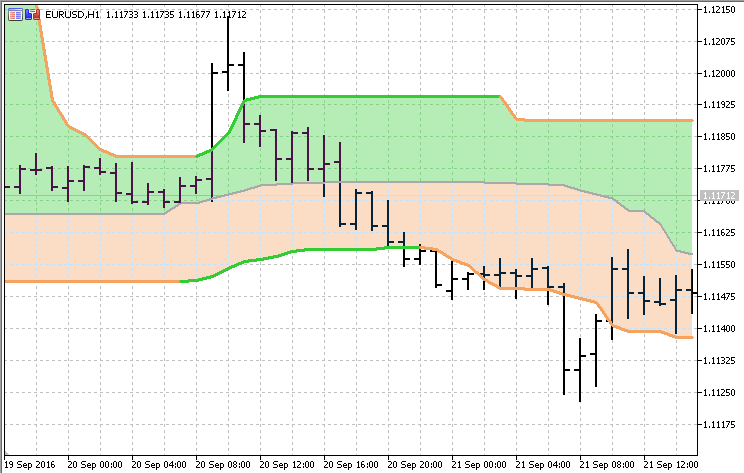Interesting script?
So post a link to it -
let others appraise it
You liked the script? Try it in the MetaTrader 5 terminal# Quantile Bands - indicator for MetaTrader 5

Views:
7466
Rating:
Published:
2016.10.04 13:43
Updated:
2017.05.02 17:07

Some basic information on quantiles :

In statistics and the theory of probability, quantiles are cutpoints dividing the range of a probability distribution into contiguous intervals with equal probabilities, or dividing the observations in a sample in the same way. There is one less quantile than the number of groups created. Thus quartiles are the three cut points that will divide a dataset into four equal-size groups (cf. depicted example). Common quantiles have special names: for instance quartile, decile (creating 10 groups: see below for more). The groups created are termed halves, thirds, quarters, etc., though sometimes the terms for the quantile are used for the groups created, rather than for the cut points.

q-Quantiles are values that partition a finite set of values into q subsets of (nearly) equal sizes. There are q − 1 of the q-quantiles, one for each integer k satisfying 0 < k < q. In some cases the value of a quantile may not be uniquely determined, as can be the case for the median (2-quantile) of a uniform probability distribution on a set of even size. Quantiles can also be applied to continuous distributions, providing a way to generalize rank statistics to continuous variables. When the cumulative distribution function of a random variable is known, the q-quantiles are the application of the quantile function (the inverse function of the cumulative distribution function) to the values {1/q, 2/q, …, (q − 1)/q}.

This indicator is constructing "bands" using 3 quantile values.Translated from Russian by MetaQuotes Ltd.
Original code: https://www.mql5.com/ru/code/16491Bollinger Bands with Filling

This version of Bollinger Bands has a choice of calculating the standard deviation as a sample or as an uncorrected deviation.Fibonacci Auto Channel

The indicator is finding out the minimum and maximum for the desired period and draws fibo zones in those bounds.New Bar Alerter

Indicator sends the alert when new bar is formed.MA Ribbon

The indicator shows the areas, where fast MA and slow MA cross.